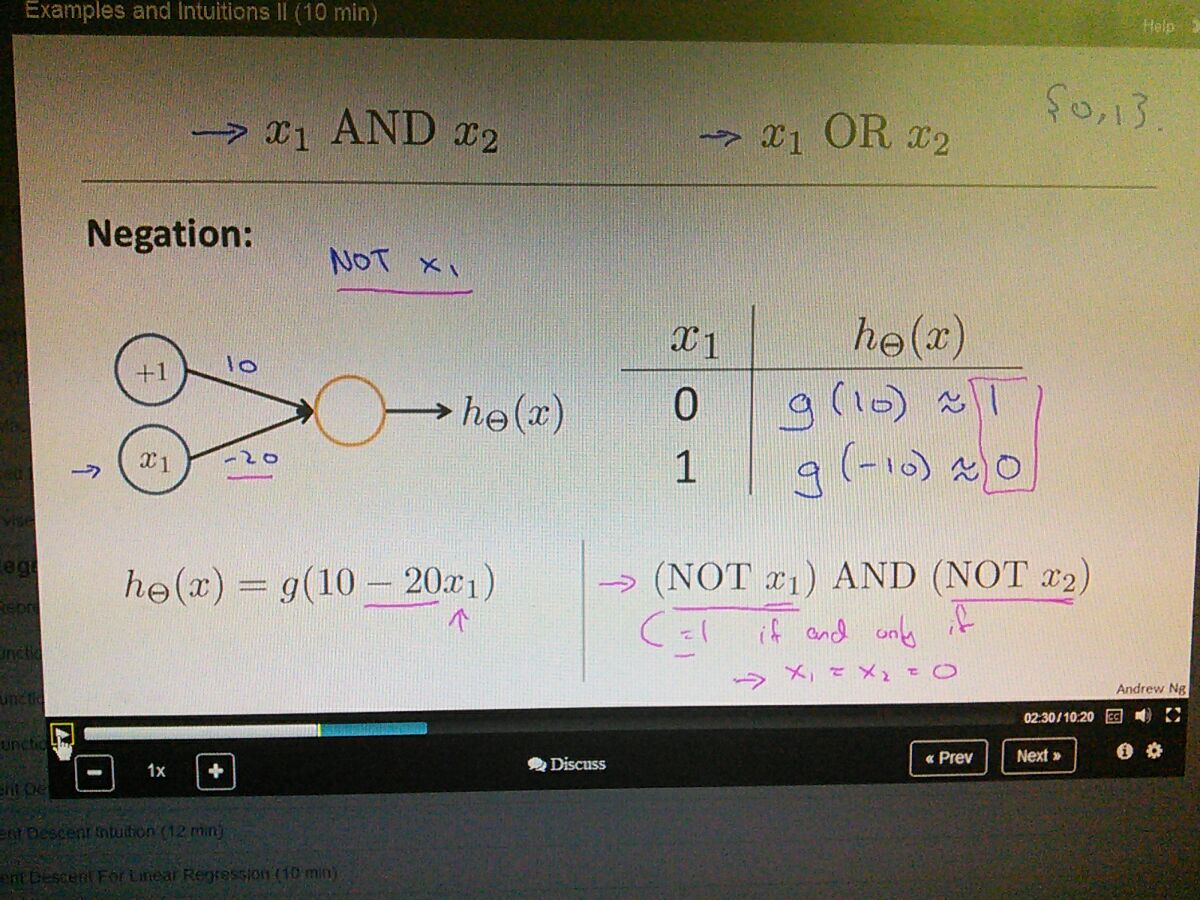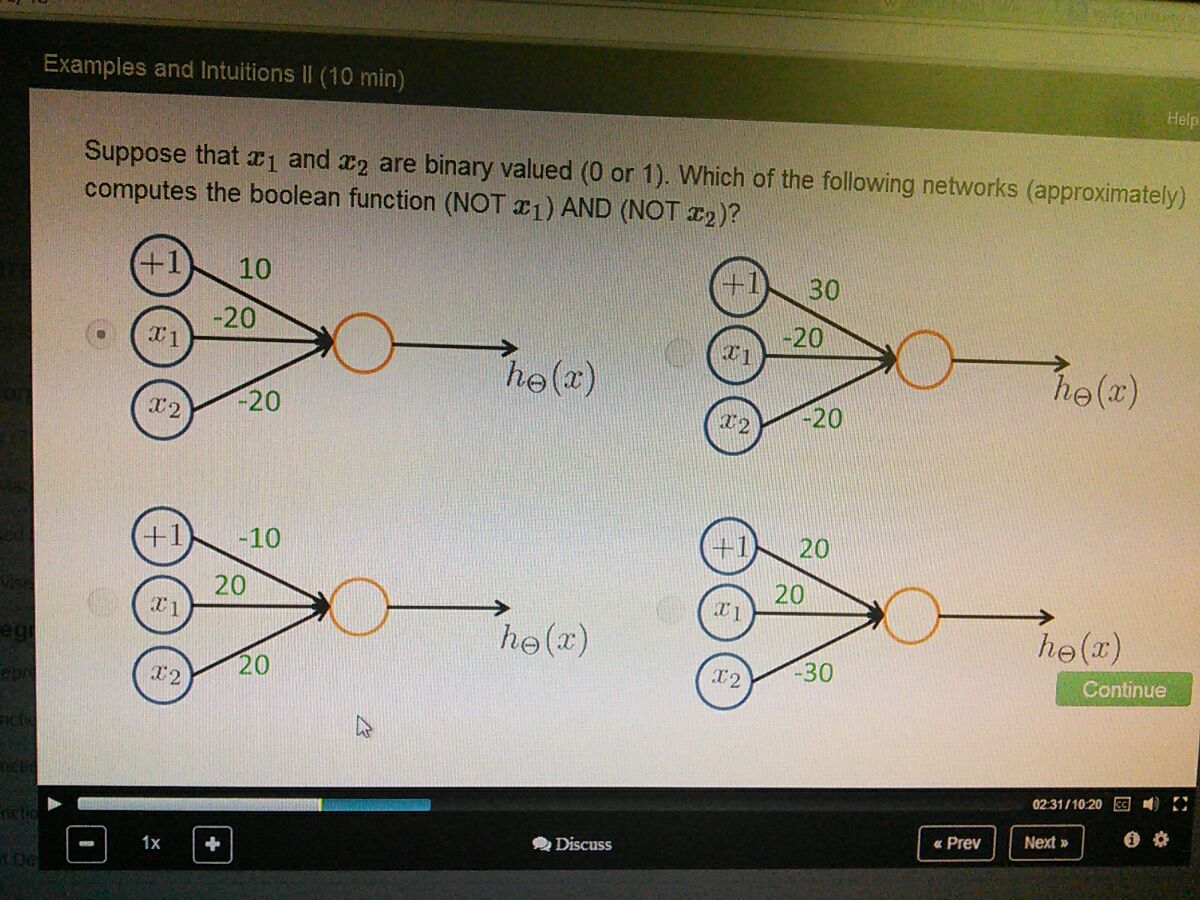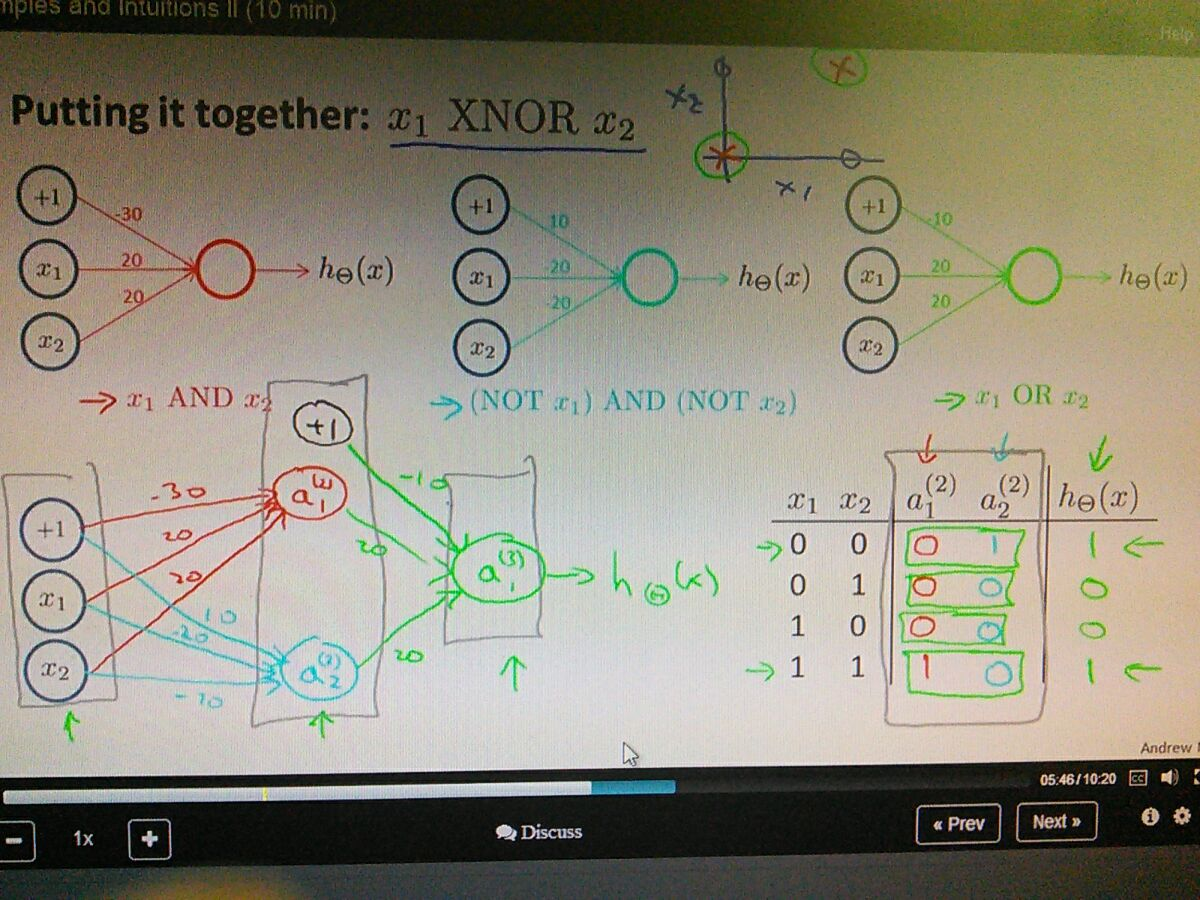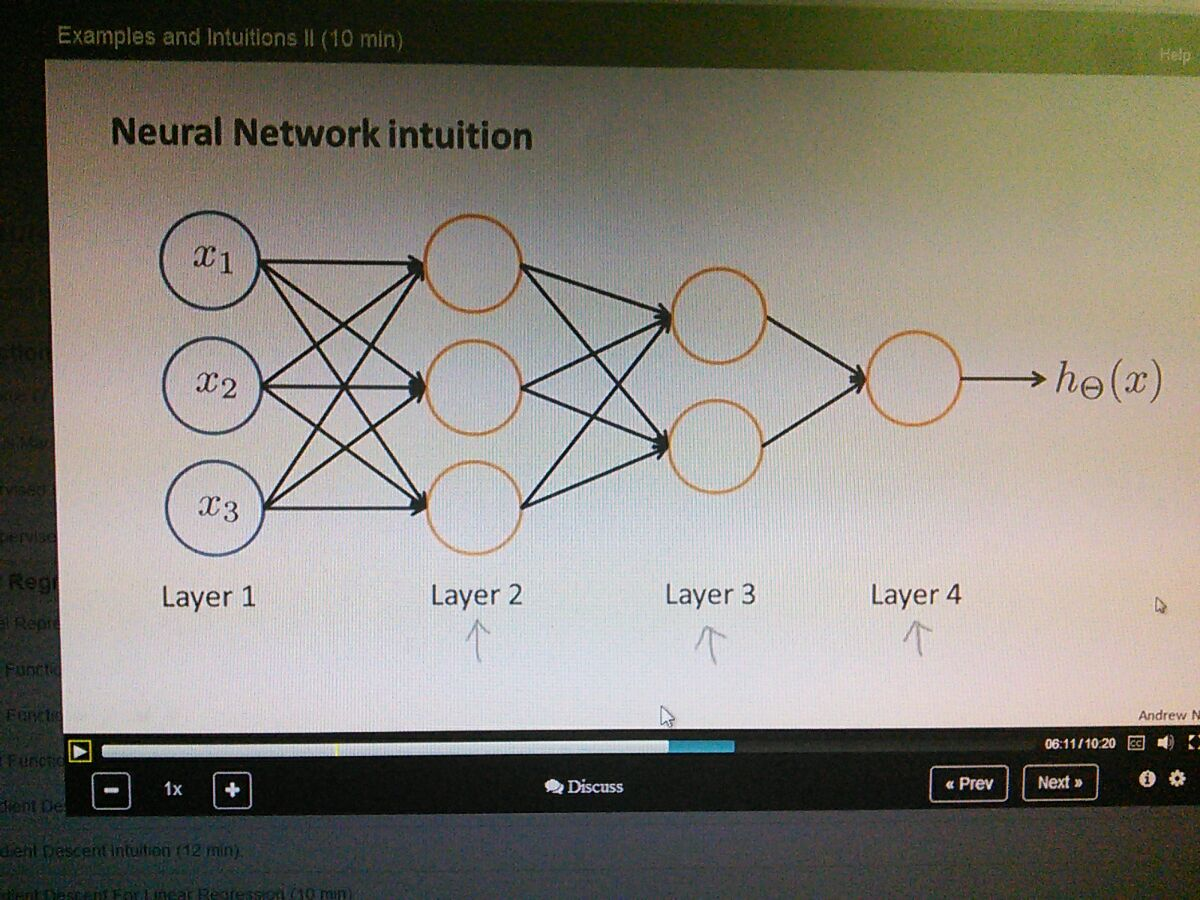• how neural networks can compute non-linear hypothesis• example of negation
• put large number to negate each other• 0 0 = - 30(1)
• 0 1 = 10(0)
• 1 0 = 10 (0)
• 1 1 = 10 (0)• xnor need non-linear decision boundaries
• xnor outputs 1 when either x1 and x2 are the same• this is why neural networks can compute more and more complicated function